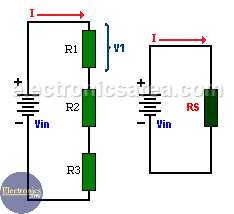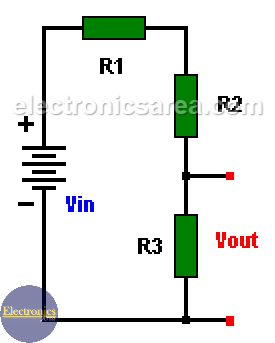# What is a Voltage divider?

The Voltage divider is a circuit that allows us to obtain an output voltage less or equal to the input voltage. The output voltage is normally obtained across ground and the resistor connected to it, but it could be across any of the other resistors.

## How a Voltage divider works?

When two or more resistors are connected in series, the current that flows through them is the same. The equivalent resistor in series is obtained adding all the resistors values. Current in the circuit is obtained using the Ohm’s Law: I = V / Rs, where Rs = R1 + R2 + R3

See the image below with the original circuit and equivalent circuit of a three resistors in series.To find out what the voltage on any of the resistor of the circuit is, we use a voltage divider formula. We know that on a series resistor circuit the current is the same for all the resistors, so using the Ohm’s Law for each resistor we find the following formulas:

• I = Vin / Rs
• I = V1 / R1
• I = V2 / R2
• I = V3 / R3

These currents are the same so, Vin / Rs = V1/R1  = V2/R2 = V3/R3Assuming that the voltage we want to know is V1, we clear this value. V1 = (Vin)(R1)/Rs. We can find V2 and V3 in the same way, using the corresponding resistor value.

## Voltage Divider Rule

Vout = (Rout/Rt) x Vin, where:

• Rout = Resistance across the output
• Rt = Total resistance of the circuit

Vout = (Resistance across the output / Total resistance of the circuit) x Vin.

## Voltage divider Examples

Using the following values: R1 = 1K, R2 = 2K, R3 = 3K and Vin = 12 volts.

1. If we want to know the voltage across resistor R3.

• Vout = Vin x R3 / ( R1 + R2 + R3)
• Vout = 12V x 3K / (1K + 2K + 3K)
• Vout = 12V x 3K / 6K = 12V / 2 = 6V

2. If we want to know the voltage cross resistor R1.

• Vout = Vin x R1 / ( R1 + R2 + R3)
• Vout = 12V x 1K / (1K + 2K + 3K)
• Vout = 12V x 1K / 6K = 12V / 6 = 2V
•
•
•
•
•
•
•
•
•
•
•
•
•
•
•
•
•
•